## Vector Norm

Based on Pythagorean Theorem, the vector from the origin to the point (3, 4) in 2D Euclidean plane has length of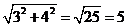and the vector from the origin to the point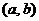has length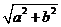. The length of a vector with two elements is the square root of the sum of each element squared.

The magnitude of a vector is sometimes called the length of a vector, or norm of a vector. Basically, norm of a vector is a measure of distance, symbolized by double vertical bar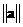.
The magnitude of a vector can be extended to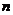dimensions. A vector a withelements has length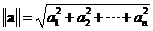The vector length is called Euclidean length or Euclidean norm. Mathematician often used term norm instead of length. Vector norm is defined as any function that associated a scalar with a vector and obeys the three rules below

1. Norm of a vector is always positive or zero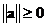. The norm of a vector is zero if and only if the vector is a zero vector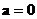.
2. A scalar multiple to a norm is equal to the product of the absolute value of the scalar and the norm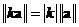.
3. Norm of a vector obeys triangular inequality that the norm of a sum of two vectors is less than or equal to the sum of the norms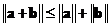.

There are many common norms:

• 1-norm is defined by the sum of absolute value of the vector elements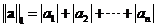.
• 2-norm is the most often used vector norm, sometimes called Euclidean norm. When the subscript index of the vector norm is not specified, you may think that it is a Euclidean norm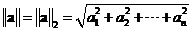.
• p-norm is sometimes called Minskowski norm is defined as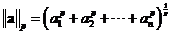. p-norm is generalized norm with a parameter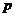.
• max-norm is also called Chebyshev norm is the largest absolute element in the vector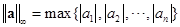.

Use the interactive program below to experiment with your own vector input. The program will give you the norm of vector for p=1, 2, 3 and max. The vector input will be redrawn to give you feedback on what you type. Click Random Example button to generate random vector.

order

## Properties

Some important properties of vector norm are

• Square of Euclidean norm is equal to the sum of square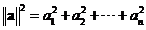.
• Pythagorean Theorem is hold if and only if the two vectors are orthogonal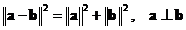.
• The law of cosine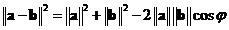• Norm of the dot product of two vectors is equal to the product of their norms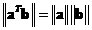.
• Relationship to vector inner products
• Square of Euclidean norm of a vector is equal to the inner product to itself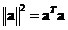•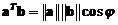where,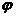is the angle between the two vectors
•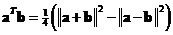• Norm of addition or subtraction follow the law of cosine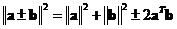• Addition of two square of norm of vectors follow parallelogram law• p-norm is greater than the max-norm but less than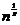times the max-norm, that is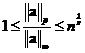.
• The norm ratio satisfies the inequality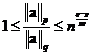. As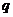tends to infinity, the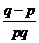approaches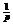and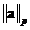approaches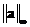.
• Cauchy-Schwartz inequality stated that the absolute value of vector dot product is always less than or equal to the product of their norms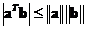. The equality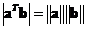holds if and only if the vectors are linearly dependent.
• Relationship of norm of cross product and dot product is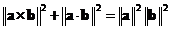.